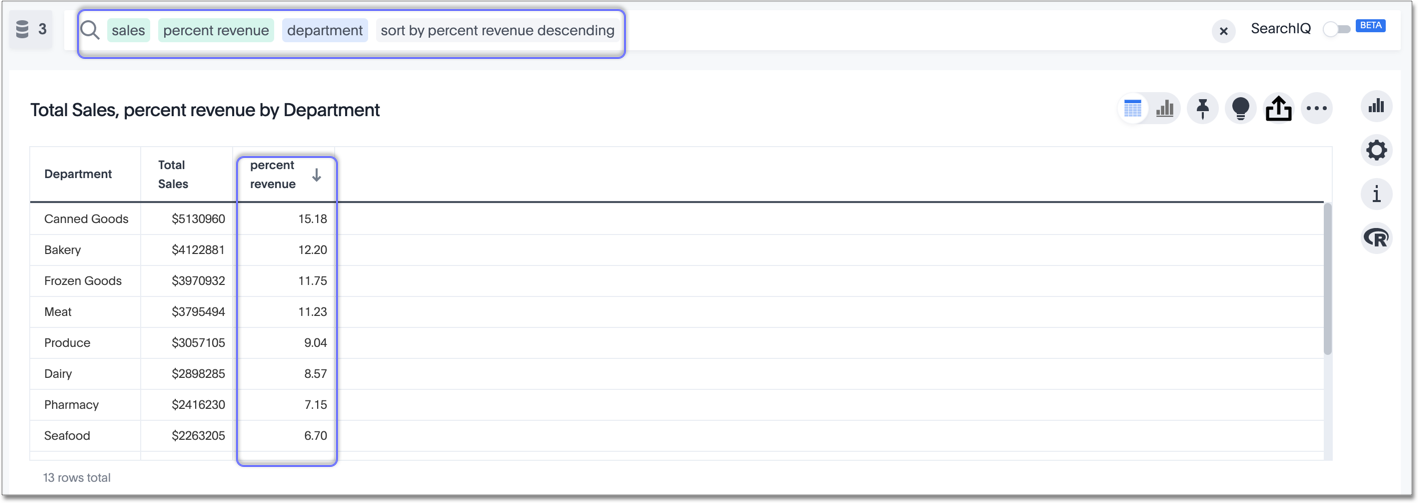# Percent (simple number) calculations

You can use simple number functions to perform useful percent calculations on your data. Simple number functions include addition, subtraction, multiplication, and division.

 * Multiplication; returns the result of multiplying two numbers. `3 * 2 = 6` `price * taxrate` + Addition; returns the result of adding two numbers. `1 + 2 = 3` `price + shipping` - Subtraction; returns the result of subtracting the second number from the first. `3 - 2 = 1` `revenue - tax` / Division; returns the result of dividing the first number by the second. `6 / 3 = 2` `markup / retail price`

## Calculate percentages

Calculating percentages is useful when you want to see, for example, the percentage of revenue that each retail department generates (gifts, bakery, canned goods, etc.).

Create a formula called `Percent Revenue` in the Formula Assistant:

`( sum ( revenue ) / group_sum ( revenue ) ) * 100`

Replace `revenue` with your company’s name for their `revenue` column, such as `revenue_dollar_amount`.

Now you can see the percentage of your total revenue that each department contributes, using the percent revenue formula that you just created. See the following example.